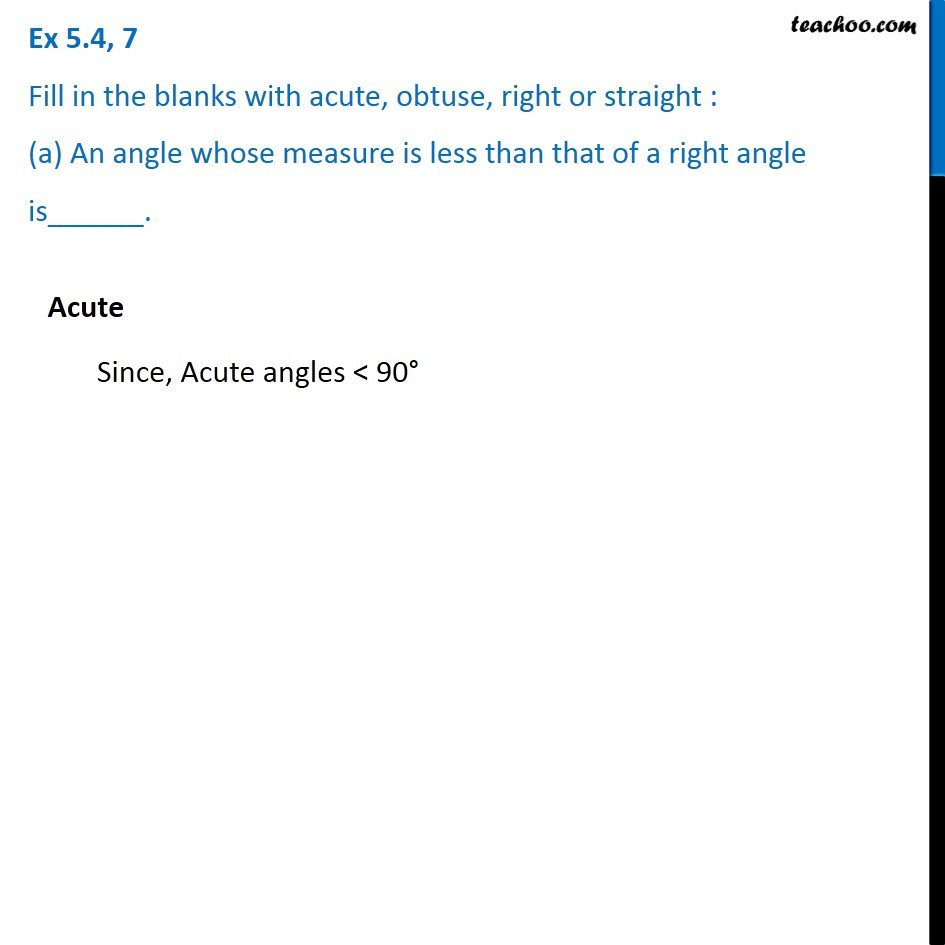1. Chapter 5 Class 6 Understanding Elementary Shapes
2. Concept wise
3. Measuring angles

Transcript

Ex 5.4, 7 Fill in the blanks with acute, obtuse, right or straight : (a) An angle whose measure is less than that of a right angle is______. Acute Since, Acute angles < 90° Ex 5.4, 7 Fill in the blanks with acute, obtuse, right or straight : (b) An angle whose measure is greater than that of a right angle is ______. Obtuse Since, Obtuse angles > 90° Ex 5.4, 7 Fill in the blanks with acute, obtuse, right or straight : (c) An angle whose measure is the sum of the measures of two right angles is _____. Straight Sum of two right angle = 90° + 90° = 180° And straight angle = 180° Ex 5.4, 7 Fill in the blanks with acute, obtuse, right or straight : (d) When the sum of the measures of two angles is that of a right angle, then each one of them is ______. Suppose two angles are ∠ A & ∠B Now , Sum of angles = 90° ∠A + ∠B = 90° So , ∠A < 90° & ∠B < 90° ∴ ∠A & ∠B are acute. Example 10° + 80° = 90 ° 20° + 70° = 90 ° 43° + 47° = 90° Ex 5.4, 7 Fill in the blanks with acute, obtuse, right or straight : (e) When the sum of the measures of two angles is that of a straight angle and if one of them is acute then the other should be _______. Suppose two angle are ∠ A & ∠ B Now , ∠ A + ∠ B = 180 Given that , One angle is acute i.e. ∠ A > 90° So , ∠ B must be greater than 90° ∴ ∠ B > 90° So , ∠ B is obtuse Example 20° + 160° =180° 80° + 100° = 180° 60° + 120° = 180°